# 12. Processing Data in Groups

The online version of the open-access textbook Minimalist Data Wrangling with Python by Marek Gagolewski is, and will remain, freely available for everyone’s enjoyment (also in PDF). Any bug/typos reports/fixes are appreciated. Although available online, this is a whole course; it should be read from the beginning to the end. In particular, refer to the Preface for general introductory remarks.

Let us consider (once again) a subset of the US Centres for Disease Control and Prevention National Health and Nutrition Examination Survey data, this time carrying some body measures (P_BMX) and demographics (P_DEMO).

nhanes = pd.read_csv("https://raw.githubusercontent.com/gagolews/" +
"teaching_data/master/marek/nhanes_p_demo_bmx_2020.csv",
comment="#")
nhanes = (
nhanes
.loc[
(nhanes.loc[:, "DMDBORN4"] <= 2) & (nhanes.loc[:, "RIDAGEYR"] >= 18),
["RIDAGEYR", "BMXWT", "BMXHT", "BMXBMI", "RIAGENDR", "DMDBORN4"]
]
.rename({
"RIDAGEYR": "age",
"BMXWT": "weight",
"BMXHT": "height",
"BMXBMI": "bmival",
"RIAGENDR": "gender",
"DMDBORN4": "usborn"
}, axis=1)
.dropna()
.reset_index(drop=True)
)

nhanes.loc[:, "usborn"] = nhanes.loc[:, "usborn"].astype("category").\
cat.rename_categories(["yes", "no"]).astype("str")

nhanes.loc[:, "gender"] = nhanes.loc[:, "gender"].astype("category").\
cat.rename_categories(["male", "female"]).astype("str")

nhanes.loc[:, "bmicat"] = pd.cut(nhanes.loc[:, "bmival"],
bins=[0, 18.5, 25, 30, np.inf],
labels=["underweight", "normal", "overweight", "obese"]
)

##    age  weight  height  bmival  gender usborn      bmicat
## 0   29    97.1   160.2    37.8  female     no       obese
## 1   49    98.8   182.3    29.7    male    yes  overweight
## 2   36    74.3   184.2    21.9    male    yes      normal
## 3   68   103.7   185.3    30.2    male    yes       obese
## 4   76    83.3   177.1    26.6    male    yes  overweight


We consider only the adult (at least 18 years old) participants, whose country of birth (the US or not) is well-defined.

We have a mix of categorical (gender, US born-ness, BMI category) and numerical (age, weight, height, BMI) variables. Unless we had encoded qualitative variables as integers, this would not be possible with plain matrices.

In this section, we will treat the categorical columns as grouping variables, so that we can, e.g., summarise or visualise the data in each group separately, because it is likely that data distributions vary across different factor levels. This is much like having many data frames stored in one object.

nhanes is thus an example of heterogeneous data at their best.

## 12.1. Basic Methods

DataFrame and Series objects are equipped with the groupby methods, which assist in performing a wide range of popular operations in data groups defined by one or more data frame columns (compare [Wic11]).

They return objects of class DataFrameGroupBy and SeriesGroupby:

type(nhanes.groupby("gender"))
## <class 'pandas.core.groupby.generic.DataFrameGroupBy'>
type(nhanes.groupby("gender").height)  # or (...)["height"]
## <class 'pandas.core.groupby.generic.SeriesGroupBy'>


Important

DataFrameGroupBy and SeriesGroupBy inherit from (extend) the GroupBy class, hence they have many methods in common. Knowing that they are separate types is useful in exploring the list of possible methods and slots in the pandas manual.

Exercise 12.1

Skim through the documentation of the said classes.

For example, the size method determines the number of observations in each group:

nhanes.groupby("gender").size()
## gender
## female    4514
## male      4271
## dtype: int64


This returns an object of type Series.

Another example, this time with grouping with respect to a combination of levels in two qualitative columns:

nhanes.groupby(["gender", "bmicat"]).size()
## gender  bmicat
## female  underweight      93
##         normal         1161
##         overweight     1245
##         obese          2015
## male    underweight      65
##         normal         1074
##         overweight     1513
##         obese          1619
## dtype: int64


This yielded a Series with a hierarchical index (row labels). We can always use reset_index to convert it to standalone columns:

nhanes.groupby(["gender", "bmicat"]).size().rename("counts").reset_index()
##    gender       bmicat  counts
## 0  female  underweight      93
## 1  female       normal    1161
## 2  female   overweight    1245
## 3  female        obese    2015
## 4    male  underweight      65
## 5    male       normal    1074
## 6    male   overweight    1513
## 7    male        obese    1619


Take note of the rename part thanks to which we have obtained a readable column name.

Exercise 12.2

Unstack the above data frame (i.e., convert it from the long to the wide format).

Exercise 12.3

(*) Note the difference between pandas.GroupBy.count and pandas.GroupBy.size methods.

### 12.1.1. Aggregating Data in Groups

The DataFrameGroupBy and SeriesGroupBy classes are equipped with a number of well-known aggregation functions, for example:

nhanes.groupby("gender").mean().reset_index()
##    gender        age     weight      height     bmival
## 0  female  48.956580  78.351839  160.089189  30.489189
## 1    male  49.653477  88.589932  173.759541  29.243620


The arithmetic mean was computed only on numeric columns. Further, a few common aggregates are generated by describe:

nhanes.groupby("gender").height.describe().reset_index()
##    gender   count        mean       std  ...    25%    50%    75%    max
## 0  female  4514.0  160.089189  7.035483  ...  155.3  160.0  164.8  189.3
## 1    male  4271.0  173.759541  7.702224  ...  168.5  173.8  178.9  199.6
##
## [2 rows x 9 columns]


But we can always apply a custom list of functions using aggregate:

(
nhanes.
loc[:, ["gender", "height", "weight"]].
groupby("gender").
aggregate([np.mean, np.median, len]).
reset_index()
)
##    gender      height                  weight
##                  mean median   len       mean median   len
## 0  female  160.089189  160.0  4514  78.351839   74.1  4514
## 1    male  173.759541  173.8  4271  88.589932   85.0  4271


The result’s columns slot is a hierarchical index.

Own functions can of course be passed as well, e.g., arbitrary inline lambda expressions:

(
nhanes.
loc[:, ["gender", "height", "weight"]].
groupby("gender").
aggregate(lambda x: (np.max(x)-np.min(x))/2).
reset_index()
)
##    gender  height  weight
## 0  female    29.1  110.85
## 1    male    27.5  102.90


Note

(**) The column names in the output object are generated by reading the applied functions’ __name__ slots, see, e.g., print(np.mean.__name__).

mr = lambda x: (np.max(x)-np.min(x))/2
mr.__name__ = "midrange"
(
nhanes.
loc[:, ["gender", "height", "weight"]].
groupby("gender").
aggregate([np.mean, mr]).
reset_index()
)
##    gender      height              weight
##                  mean midrange       mean midrange
## 0  female  160.089189     29.1  78.351839   110.85
## 1    male  173.759541     27.5  88.589932   102.90


### 12.1.2. Transforming Data in Groups

We can easily transform individual columns relative to different data groups by means of the transform method for GroupBy objects.

def standardise(x):
return (x-np.mean(x, axis=0))/np.std(x, axis=0, ddof=1)

nhanes["height_std"] = (
nhanes.
loc[:, ["height", "gender"]].
groupby("gender").
transform(standardise)
)
(
nhanes.
loc[:, ["gender", "height", "height_std"]].
groupby("gender").
aggregate([np.mean, np.std])
)
##             height              height_std
##               mean       std          mean  std
## gender
## female  160.089189  7.035483 -1.353518e-15  1.0
## male    173.759541  7.702224  3.155726e-16  1.0


The new column gives the relative z-scores: a woman with relative z-score of 0 has height of 160.1 cm, whereas a man with the same z-score has height of 173.8 cm.

Exercise 12.4

Create a data frame comprised of 5 tallest men and 5 tallest women.

### 12.1.3. Manual Splitting Into Subgroups (*)

It turns out that GroupBy objects and their derivatives are iterable (compare Section 3.4), therefore the grouped data frames and series can be easily processed manually in case where the built-in methods are insufficient (i.e., not so rarely).

Let us consider a small sample of our data frame.

grouped = nhanes.loc[:5, ["gender", "weight", "height"]].groupby("gender")
list(grouped)
## [('female',    gender  weight  height
## 0  female    97.1   160.2
## 5  female    91.1   152.7), ('male',   gender  weight  height
## 1   male    98.8   182.3
## 2   male    74.3   184.2
## 3   male   103.7   185.3
## 4   male    83.3   177.1)]


Therefore, when iterating through a GroupBy object, we get access to pairs giving all the levels of the grouping variable and the subsets of the input data frame corresponding to these categories.

for level, df in grouped:
# df is a data frame - we can do whatever we want
print(f"There are {df.shape} subjects with gender={level}.")
## There are 2 subjects with gender=female.
## There are 4 subjects with gender=male.


Let us also demonstrate that the splitting can be done manually without the use of pandas. Calling numpy.split(arr, ind) returns a list with arr (being an array-like object, e.g., a matrix, a vector, or a data frame) split rowwisely into len(ind)+1 chunks at indices given by ind.

For example:

np.split(np.arange(10)*10, [3, 7])
## [array([ 0, 10, 20]), array([30, 40, 50, 60]), array([70, 80, 90])]


To split a data frame into groups defined by a categorical column, we can first sort it with respect to the criterion of interest, for instance, the gender data:

nhanes_srt = nhanes.sort_values("gender", kind="stable")


Then, we can use numpy.unique to fetch the indices of first occurrences of each series of identical labels:

levels, where = np.unique(nhanes_srt.loc[:, "gender"], return_index=True)
levels, where
## (array(['female', 'male'], dtype=object), array([   0, 4514]))


This can now be used for dividing the sorted data frame into chunks:

nhanes_grp = np.split(nhanes_srt, where[1:])


We obtained a list of data frames split at rows specified by where[1:]. Here is a preview of the first and the last row in each chunk:

for i in range(len(levels)):
print(f"level='{levels[i]}'; preview:")
print(nhanes_grp[i].iloc[ [0, -1], : ])
print("")
## level='female'; preview:
##       age  weight  height  bmival  gender usborn bmicat  height_std
## 0      29    97.1   160.2    37.8  female     no  obese    0.015750
## 8781   67    82.8   147.8    37.9  female     no  obese   -1.746744
##
## level='male'; preview:
##       age  weight  height  bmival gender usborn      bmicat  height_std
## 1      49    98.8   182.3    29.7   male    yes  overweight    1.108830
## 8784   74    59.7   167.5    21.3   male     no      normal   -0.812693


We can apply any operation on each subgroup we have learned so far, our imagination is the only limiting factor, e.g., aggregate some columns:

nhanes_agg = [
dict(
level=t.loc[:, "gender"].iloc,
height_mean=np.mean(t.loc[:, "height"]),
weight_mean=np.mean(t.loc[:, "weight"])
)
for t in nhanes_grp
]
nhanes_agg
## [{'level': 'female', 'height_mean': 160.0891891891892, 'weight_mean': 78.35183872396988}, {'level': 'male', 'height_mean': 173.75954109107937, 'weight_mean': 88.58993210021072}]


Finally, the results may be combined, e.g., to form a data frame:

pd.DataFrame(nhanes_agg)
##     level  height_mean  weight_mean
## 0  female   160.089189    78.351839
## 1    male   173.759541    88.589932


We see that manual splitting is very powerful but quite tedious in case where we would like to perform the basic operations such as computing some basic aggregates. These scenarios are very common, no wonder why the pandas developers came up with an additional, convenience interface in the form of the pandas.DataFrame.groupby and pandas.Series.groupby methods and the DataFrameGroupBy and SeriesGroupby classes. However, it is still worth knowing the low-level way to perform splitting in case of more ambitious tasks.

Exercise 12.5

(**) Using numpy.split and matplotlib.pyplot.boxplot, draw a box-and-whisker plot of heights grouped by BMI category (four boxes side by side).

Exercise 12.6

(**) Using numpy.split, compute the relative z-scores of the height column separately for each BMI category.

Note

(**) A simple trick to allow grouping with respect to more than one column is to apply numpy.unique on a string vector that combines the levels of the grouping variables, e.g., by concatenating them like nhanes_srt.gender + "___" + nhanes_srt.bmicat (assuming that nhanes_srt is ordered with respect to these two criteria).

## 12.2. Plotting Data in Groups

The seaborn package is particularly convenient for plotting grouped data – it is highly interoperable with pandas.

### 12.2.1. Series of Box Plots

For example, Figure 12.1 depicts a box plot with four boxes side by side:

sns.boxplot(x="bmival", y="gender", hue="usborn",
data=nhanes, palette="Set2")
plt.show()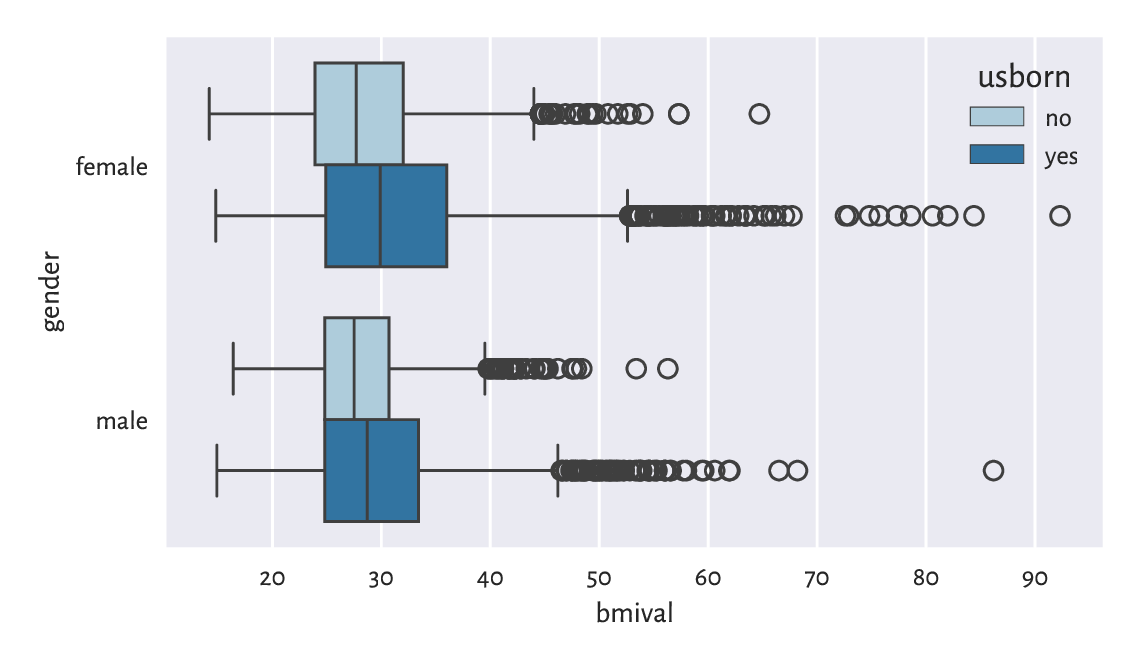Figure 12.1 The distribution of BMIs for different genders and countries of birth

Let us contemplate for a while how easy it is now to compare the BMI distribution in different groups. Here, we have two grouping variables, as specified by the y and hue arguments.

Exercise 12.7

Create a similar series of violin plots.

Exercise 12.8

Add the average BMIs in each group to the above box plot.

### 12.2.2. Series of Bar Plots

In Figure 12.2, on the other hand, we have a bar plot representing a two way contingency table (obtained in a different way than in Chapter 11):

sns.barplot(
y="counts",
x="gender",
hue="bmicat",
palette="Set2",
data=(
nhanes.
groupby(["gender", "bmicat"]).
size().
rename("counts").
reset_index()
)
)
plt.show()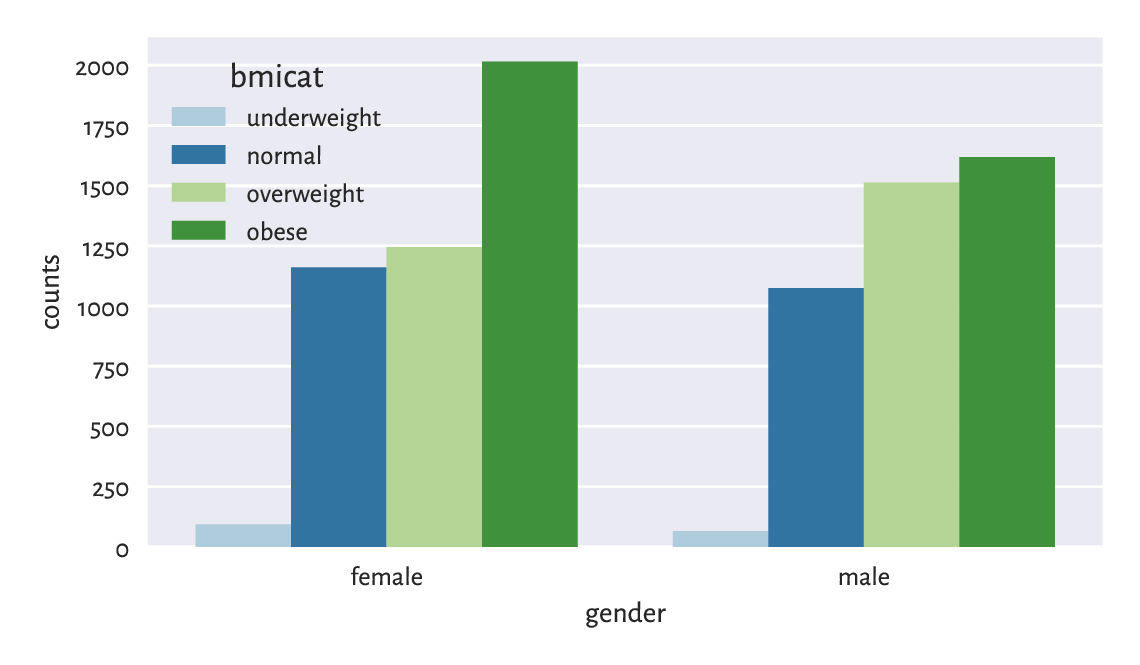Figure 12.2 Number of persons for each gender and BMI category

Exercise 12.9

Draw a similar bar plot where the bar heights sum to 100% for each gender.

Exercise 12.10

Using the two-sample chi-squared test, verify whether the BMI category distributions differ significantly from each other across genders.

### 12.2.3. Semitransparent Histograms

Figure 12.3 illustrates that playing with semitransparent objects can make comparisons easy. By passing common_norm=False, we have scaled each density histogram separately, which is the behaviour we desire is samples are of different lengths.

sns.histplot(data=nhanes, x="weight", hue="usborn",
element="step", stat="density", common_norm=False)
plt.show()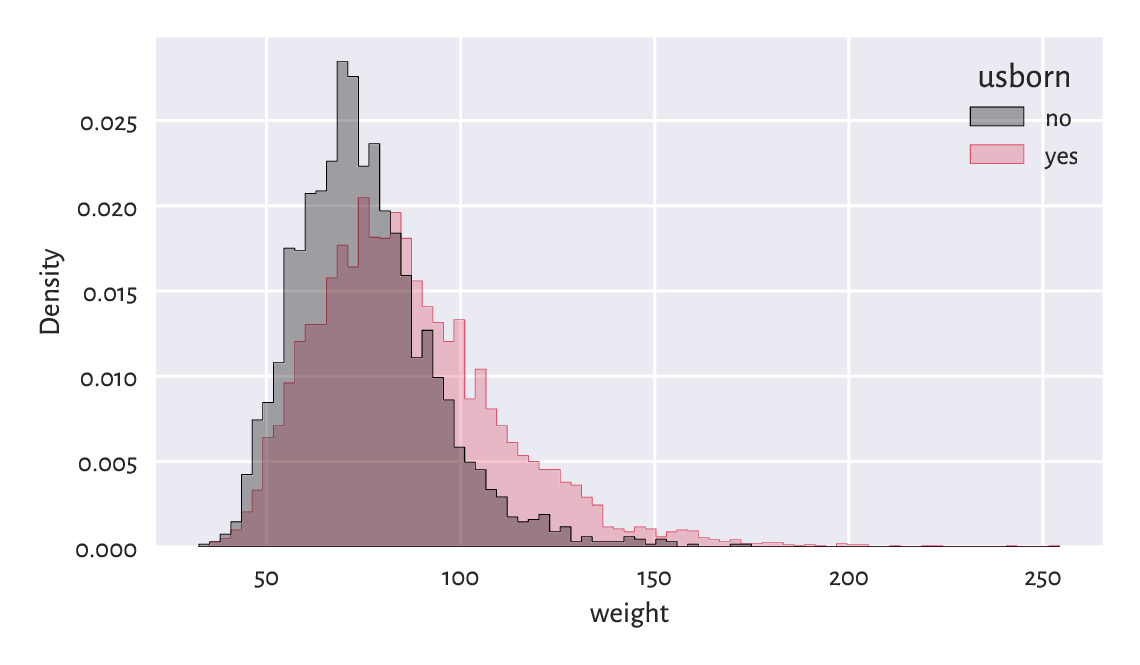Figure 12.3 Clearly, the weight distribution of the US-born participants has higher mean and variance

### 12.2.4. Scatterplots with Group Information

Scatterplots for grouped data can display category information using points of different shapes or colours, compare Figure 12.4.

sns.scatterplot(x="height", y="weight", hue="gender", data=nhanes, alpha=0.1)
plt.show()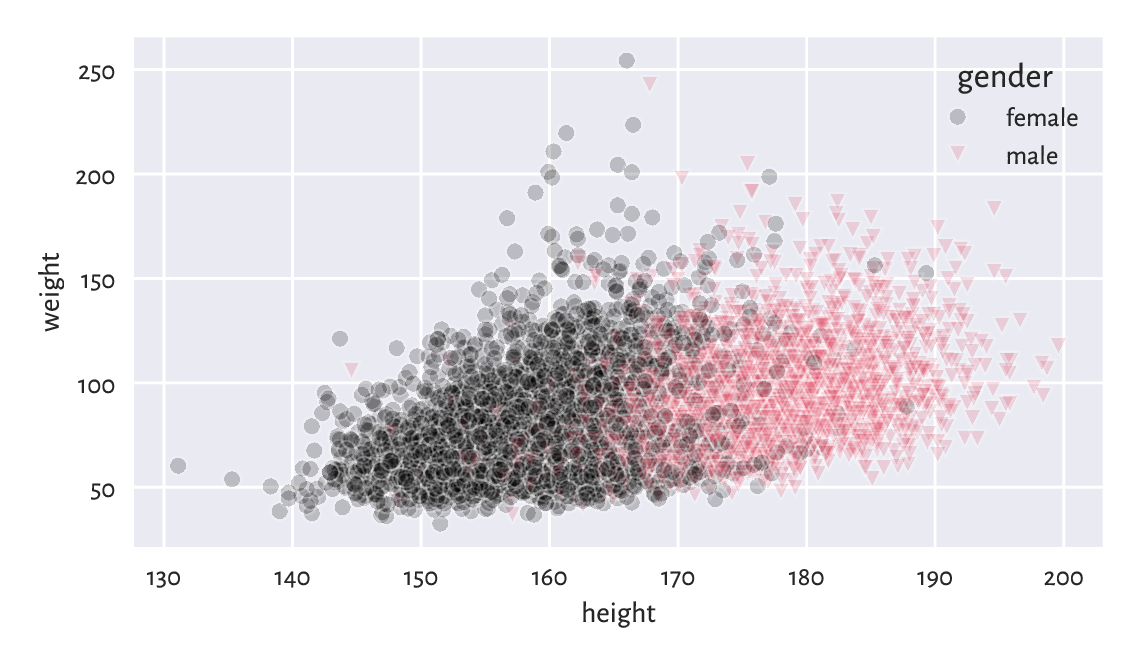Figure 12.4 Weight vs height grouped by gender

### 12.2.5. Grid (Trellis) Plots

Grid plot (also known as trellis, panel, or lattice plots) are a way to visualise data separately for each factor level. All the plots share the same coordinate ranges which makes them easily comparable. For instance, Figure 12.5 depicts a series of histograms of weights grouped by a combination of two categorical variables.

grid = sns.FacetGrid(nhanes, col="gender", row="usborn")
grid.map(sns.histplot, "weight", stat="density", color="lightgray")
# plt.show()  # not required...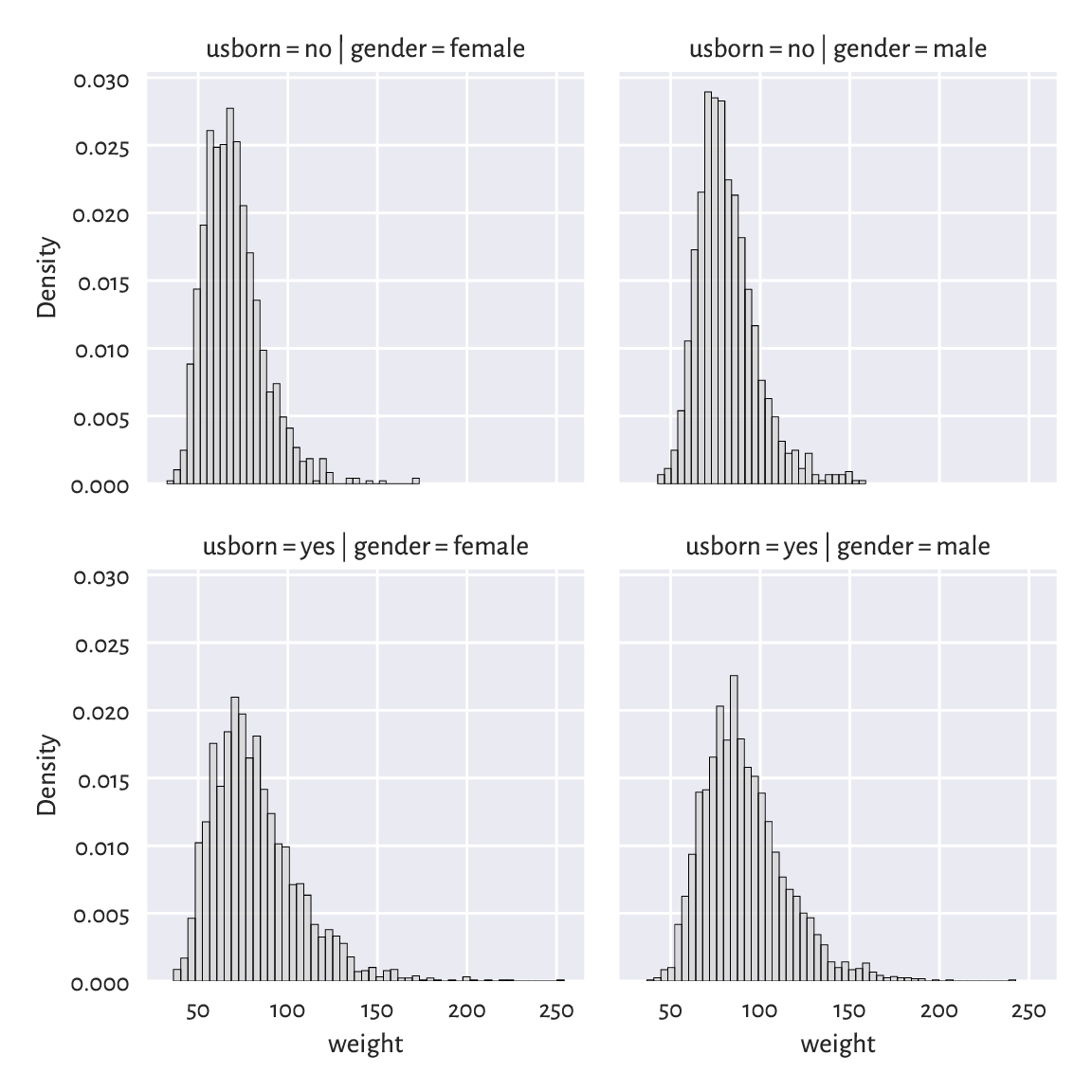Figure 12.5 Distribution of weights for different genders and countries of birth

Exercise 12.11

Pass hue="bmicat" additionally to seaborn.FacetGrid.

Important

Grid plots can bear any kind of data visualisation we have discussed so far (e.g., histograms, bar plots, scatterplots).

Exercise 12.12

Draw a trellis plot with scatterplots of weight vs height grouped by BMI category and gender.

### 12.2.6. Comparing ECDFs with the Two-Sample Kolmogorov–Smirnov Test (*)

Figure 12.6 compares the empirical cumulative distribution functions of the weight distributions for US and non-US born participants.

sns.ecdfplot(data=nhanes, x="weight", hue="usborn")
plt.show()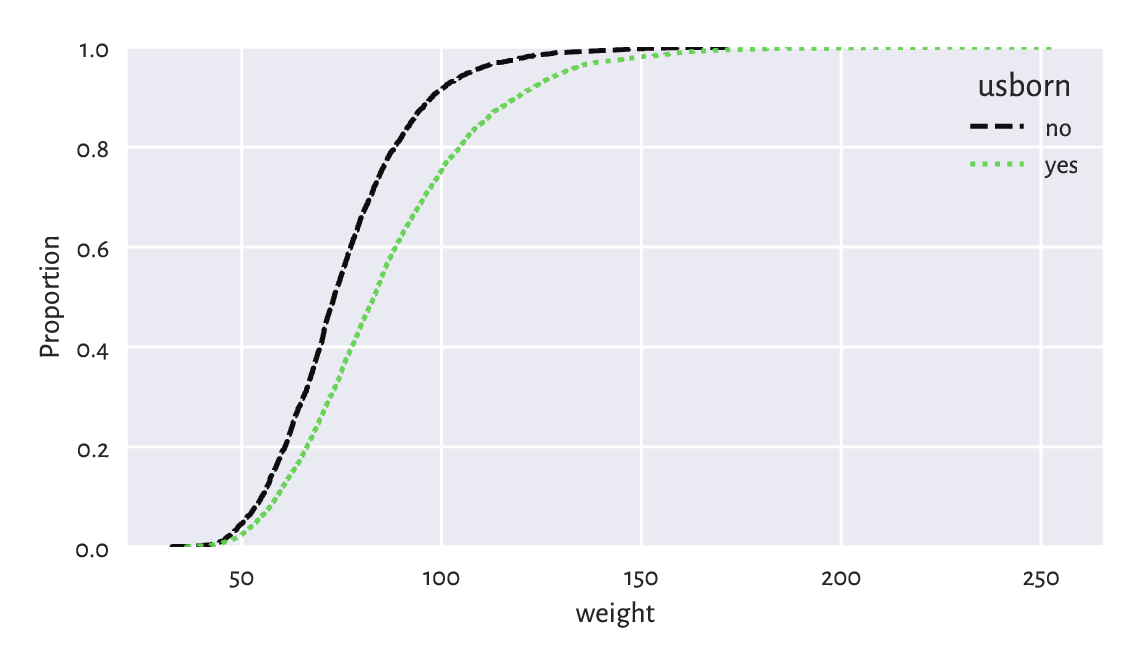Figure 12.6 Empirical culumative distribution functions of weight distributions for different birthplaces

A two-sample Kolmogorov–Smirnov test can be used to check whether two empirical cumulative distribution functions $$\hat{F}_n'$$ (e.g., weight of the US-born participants) and $$\hat{F}_m''$$ (e.g., weight of non-US-born persons) are significantly different or not from each other:

$\begin{split} \left\{ \begin{array}{rll} H_0: & \hat{F}_n' = \hat{F}_n'' & \text{(null hypothesis)}\\ H_1: & \hat{F}_n' \neq \hat{F}_n'' & \text{(two-sided alternative)} \\ \end{array} \right. \end{split}$

The test statistic is a variation of the one-sample variant discussed in Section 6.2.3. Namely, let

$\hat{D}_{n,m} = \sup_{t\in\mathbb{R}} | \hat{F}_n'(t) - \hat{F}_m''(t) |.$

Computing the above is slightly trickier than in the previous case, but luckily an appropriate procedure is already implemented in scipy:

x12 = nhanes.set_index("usborn").weight
x1 = x12.loc["yes"]
x2 = x12.loc["no"]
Dnm = scipy.stats.ks_2samp(x1, x2)
Dnm
## 0.22068075889911914


Assuming significance level $$\alpha=0.001$$, the critical value is approximately (for larger $$n$$ and $$m$$) equal to

$K_{n,m} = \sqrt{ -\frac{ \log(\alpha/2) (n+m) }{ 2nm } },$
alpha = 0.001
np.sqrt(-np.log(alpha/2) * (len(x1)+len(x2)) / (2*len(x1)*len(x2)))
## 0.04607410479813944


As usual, we reject the null hypothesis when $$\hat{D}_{n,m}\ge K_{n,m}$$, which is exactly the case here (at significance level $$0.1\%$$). In other words, weights of US- and non-US-born participants differ significantly.

Important

Frequentist hypothesis testing only takes into account the deviation between distributions that is explainable due to sampling effects (the assumed randomness of the data generation process). For large sample sizes, even very small deviations1 will be deemed statistically significant, but it does not mean that we should consider them as practically significant. For instance, if a very costly, environmentally unfriendly, and generally inconvenient for everyone upgrade leads to a process’ improvement such that we reject the null hypothesis stating that two distributions are equal, but it turns out that the gains are ca. 0.5%, the good old common sense should be applied.

Exercise 12.13

Compare ECDFs of weights of males who are between 18 and 25 years old. Determine whether they are significantly different.

Important

Some statistical textbooks and many research papers in the social sciences (amongst many others) employ the significance level of $$\alpha=5\%$$, which is often criticised as too high (and for similar reasons we do not introduce the notion of $$p$$-vales, which most practitioners misunderstand anyway). As many stakeholders aggressively push towards constant improvements in terms of inventing bigger, better, faster, more efficient things, larger $$\alpha$$ allows for generating more sensational discoveries, because it considers smaller differences as already significant, and thus possibly adding to what we call the reproducibility crisis in the empirical sciences.

We, on the other hand, claim that it is better to err on the side of being cautious – which, in the long run, is more sustainable, and eco-friendly.

### 12.2.7. Comparing Quantiles

Plotting quantiles in two samples against each other can also give us some further (informal) insight with regard to the possible distributional differences. Figure 12.7 depicts an example Q-Q plot (compare Section 6.2.2 for a one-sample version), where we see that the distributions have similar shapes (points more or less lie on a straight line), but they are shifted and/or scaled (if they were, they would be on the identity line).

x = nhanes.weight.loc[nhanes.usborn == "yes"]
y = nhanes.weight.loc[nhanes.usborn == "no"]
xd = np.sort(x)
yd = np.sort(y)
if len(xd) > len(yd):  # interpolate between quantiles in a longer sample
xd = np.quantile(xd, np.arange(1, len(yd)+1)/(len(yd)+1))
else:
yd = np.quantile(yd, np.arange(1, len(xd)+1)/(len(xd)+1))
plt.plot(xd, yd, "o")
plt.axline((xd[len(xd)//2], xd[len(xd)//2]), slope=1,
linestyle=":", color="gray")  # identity line
plt.xlabel(f"Sample quantiles (weight; usborn=yes)")
plt.ylabel(f"Sample quantiles (weight; usborn=no)")
plt.show()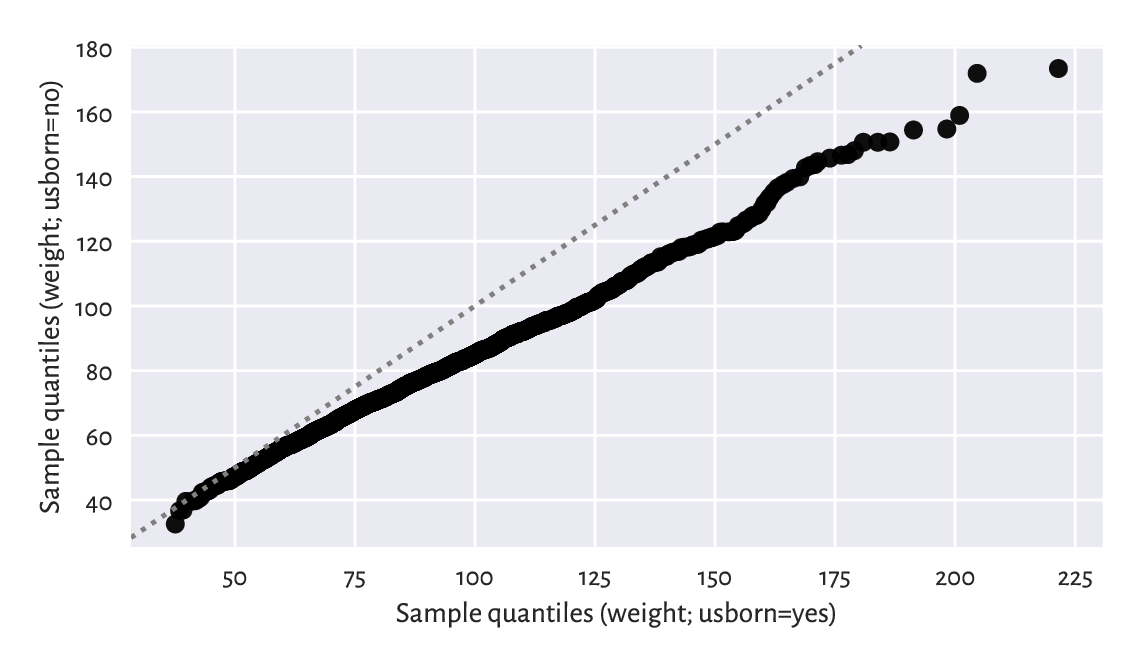Figure 12.7 A two-sample Q-Q plot

Notice that we interpolated between the quantiles in a larger sample to match the length of the shorter vector.

Let us consider a small sample of white, rather sweet wines from a much larger wine quality dataset.

wine_train = pd.read_csv("https://raw.githubusercontent.com/gagolews/" +
"teaching_data/master/other/sweetwhitewine_train2.csv",
comment="#")
## 0  10.625271  10.340159    0
## 1   9.066111  18.593274    1
## 2  10.806395   6.206685    0
## 3  13.432876   2.739529    0
## 4   9.578162   3.053025    0


We are given each wine’s alcohol and residual sugar content, as well as a binary categorical variable stating whether a group of sommeliers deem a given beverage quite bad (1) or not (0). Figure 12.8 reveals that bad wines are rather low in… alcohol and, to some extent, sugar.

sns.scatterplot(x="alcohol", y="sugar",
plt.xlabel("alcohol")
plt.ylabel("sugar")
plt.show()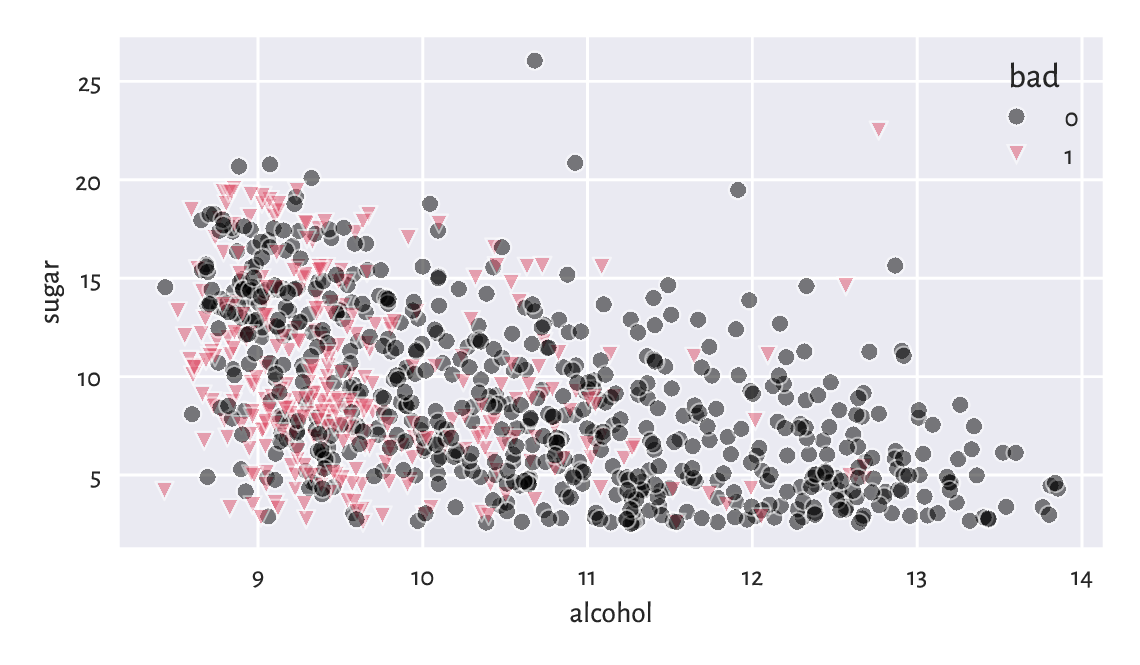Figure 12.8 Scatterplot for sugar vs alcohol content for white, rather sweet wines, and whether they are considered bad (1) or drinkable (0) by some experts

Someone answer the door! We have just received a delivery: quite a few new wine bottles whose alcohol and sugar contents have, luckily, been given on their respective labels.

wine_test = pd.read_csv("https://raw.githubusercontent.com/gagolews/" +
"teaching_data/master/other/sweetwhitewine_train2.csv",
comment="#").iloc[:, :-1]
##      alcohol      sugar
## 0  10.625271  10.340159
## 1   9.066111  18.593274
## 2  10.806395   6.206685
## 3  13.432876   2.739529
## 4   9.578162   3.053025


We would like to determine which of the wines from the test set might be not-bad without asking an expert for their opinion. In other words, we would like to exercise a classification task. More formally:

Important

Assume we are given a set of training points $$\mathbf{X}\in\mathbb{R}^{n\times m}$$ and the corresponding reference outputs $$\boldsymbol{y}\in\{L_1,L_2,\dots,L_l\}^n$$ in the form of a categorical variable with l distinct levels. The aim of a classification algorithm is to predict what the outputs for each point from a possibly different dataset $$\mathbf{X}'\in\mathbb{R}^{n'\times m}$$, i.e., $$\hat{\boldsymbol{y}}'\in\{L_1,L_2,\dots,L_l\}^{n'}$$, might be.

In other words, we are asked to fill the gaps in a categorical variable. Recall that in a regression problem (Section 9.2), the reference outputs were numerical.

Exercise 12.14

Which of the following are instances of classification problems and which are regression tasks?

• Detect email spam;

• Predict a market stock price;

• Predict the likeability of a new advertising piece;

• Assess credit risk;

• Detect tumour tissues in medical images;

• Predict time-to-recovery of cancer patients;

• Recognise smiling faces on photographs;

• Detect unattended luggage in airport security camera footage;

• Turn on emergency braking to avoid a collision with pedestrians in autonomous vehicle.

What kind of data should you gather in order to tackle them?

### 12.3.1. K-Nearest Neighbour Classification

One of the simplest approaches to classification – good enough for such an introductory course – is based on the information about a test point’s nearest neighbours (compare Section 8.4.4) living in the training sample.

Fix $$k\ge 1$$. Namely, to classify some $$\boldsymbol{x}'\in\mathbb{R}^m$$:

1. Find the indices $$N_k(\boldsymbol{y})=\{i_1,\dots,i_k\}$$ of the $$k$$ points from $$\mathbf{X}$$ closest to $$\boldsymbol{x}'$$, i.e., ones that fulfil for all $$j\not\in\{i_1,\dots,i_k\}$$

$\|\mathbf{x}_{i_1,\cdot}-\boldsymbol{x}'\| \le\dots\le \| \mathbf{x}_{i_k,\cdot} -\boldsymbol{x}' \| \le \| \mathbf{x}_{j,\cdot} -\boldsymbol{x}' \|.$
2. Classify $$\boldsymbol{x}'$$ as $$y'=\mathrm{mode}(y_{i_1},\dots,y_{i_k})$$, i.e., assign it the label that most frequently occurs amongst its $$k$$ nearest neighbours. If a mode is nonunique, resolve the ties, for example, at random.

It is thus a very similar algorithm to k-nearest neighbour regression (Section 9.2.1), where we replaced the quantitative mean with the qualitative mode.

This is a variation on the theme: if you don’t know what to do in a given situation, try to mimic what most of the other people around you are doing. Or, if you don’t know what to think about a particular wine, but amongst the 5 similar ones (in terms of alcohol and sugar content) three were said to be awful, say that you don’t like it because it’s not sweet enough. Thanks to this, others will think you are a very sophisticated wine taster.

Let us apply a 5-nearest neighbour classifier on the standardised version of the dataset: as we are about to use a technique based on pairwise distances, it would be best if the variables were on the same scale.

k = 5


Computing the z-scores for the train set:

X_train = wine_train.loc[:, ["alcohol", "sugar"]].to_numpy()
means = np.mean(X_train, axis=0)
sds = np.std(X_train, axis=0)
Z_train = (X_train-means)/sds


The z-scores for the test set (let us stress that they are based on the aggregates computed for the train set):

Z_test = (wine_test.loc[:, ["alcohol", "sugar"]].to_numpy()-means)/sds


Making the predictions:

def knn_class(X_test, X_train, y_train, k):
nnis = scipy.spatial.KDTree(X_train).query(X_test, k)
nnls = y_train[nnis]  # same as: y_train[nnis.reshape(-1)].reshape(-1, k)
return scipy.stats.mode(nnls.reshape(-1, k), axis=1).reshape(-1)

y_pred = knn_class(Z_test, Z_train, y_train, k)
y_pred[:10]  # preview
## array([0, 1, 0, 0, 1, 0, 0, 0, 0, 0])


First, we have fetched the indices of each test point’s nearest neighbours (amongst the points in the training set). Then, we have fetched their corresponding labels; they are stored in a matrix with $$k$$ columns. Finally, we computed the modes in each row, and hence classified each point in the test set.

Note

Unfortunately, scipy.stats.mode does not resolve the possible ties at random. Nevertheless, in our case, $$k$$ is odd and the number of possible classes is $$l=2$$, therefore the mode is always unique.

Figure 12.9 shows how nearest neighbour classification categorises different regions of a section of the two-dimensional plane. The greater the k, the smoother the decision boundaries. Naturally, in regions corresponding to few training points we do not expect the classification accuracy to be good enough.

x1 = np.linspace(Z_train[:, 0].min(), Z_train[:, 0].max(), 100)
x2 = np.linspace(Z_train[:, 1].min(), Z_train[:, 1].max(), 100)
xg1, xg2 = np.meshgrid(x1, x2)
Xg12 = np.column_stack((xg1.reshape(-1), xg2.reshape(-1)))
ks = [5, 25]
for i in range(len(ks)):
plt.subplot(1, len(ks), i+1)
yg12 = knn_class(Xg12, Z_train, y_train, ks[i])
plt.scatter(Z_train[:, 0], Z_train[:, 1],
c=np.array(["black", "red"])[y_train], alpha=0.5)
plt.contourf(x1, x2, yg12.reshape(len(x2), len(x1)),
cmap="RdGy_r", alpha=0.5)
plt.title(f"$k={ks[i]}$")
plt.xlabel("alcohol")
plt.ylabel("sugar")
plt.show()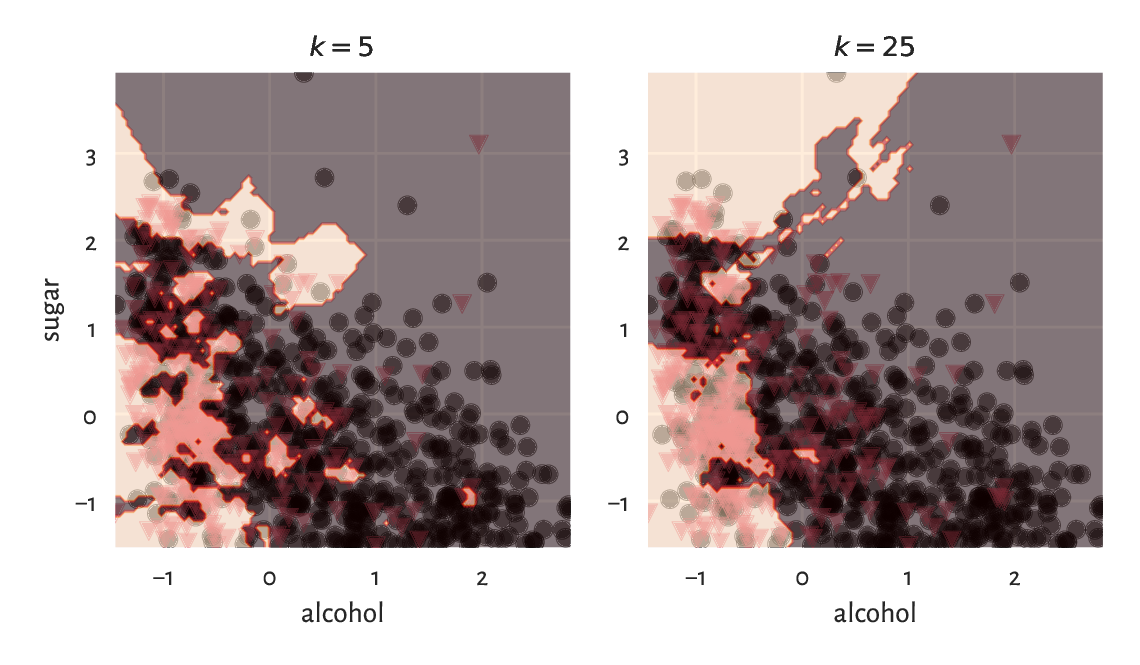Figure 12.9 K-nearest neighbour classification of a whole, dense, two-dimensional grid of points for different k

Example 12.15

(**) The same with the scikit-learn package:

import sklearn.neighbors
knn = sklearn.neighbors.KNeighborsClassifier(k)
knn.fit(Z_train, y_train)
y_pred2 = knn.predict(Z_test)


We can verify that the results are identical to the ones above by calling:

np.all(y_pred2 == y_pred)
## True


### 12.3.2. Assessing the Quality of Predictions

It is time to reveal the truth: our test wines, it turns out, have already been assessed by some experts.

y_test = pd.read_csv("https://raw.githubusercontent.com/gagolews/" +
"teaching_data/master/other/sweetwhitewine_train2.csv",
comment="#").iloc[:, -1].to_numpy()
y_test[:10]  # preview
## array([0, 1, 0, 0, 0, 1, 0, 0, 0, 1])


The accuracy score is the most straightforward measure of the similarity between these true labels (denoted $$\boldsymbol{y}'$$) and the ones predicted by the classifier (denoted $$\hat{\boldsymbol{y}}'$$). It is defined as a ratio of the correctly classified instances to all the instances.

np.mean(y_test == y_pred)
## 0.788


Thus, 79% of the wines were correctly classified with regard to their true quality. Before we get too enthusiastic, let us note that our dataset is slightly imbalanced:

pd.Series(y_test).value_counts()  # contingency table
## 0    639
## 1    361
## dtype: int64


It turns out that 639 out of 1000 wines in our sample are (truly) good. Therefore, a classifier which labels all the wines as great would have accuracy of ca. 64%. Thus, our machine learning approach to wine quality assessment is not that usable after all.

Thus, it is always good to analyse the corresponding confusion matrix, which is a two-way contingency table summarising the correct decisions and errors we make.

C = pd.DataFrame(
dict(y_pred=y_pred, y_test=y_test)
).value_counts().unstack(fill_value=0)
C
## y_test    0    1
## y_pred
## 0       548  121
## 1        91  240


In the binary classification case ($$l=2$$) such as this one, its entries are usually referred to as what is given in the table below. The terms positive and negative refer to the output predicted by a classifier, i.e., they indicate whether some $$\hat{y}'$$ is equal to 1 and 0, respectively.

Table 12.1 The different cases of true vs predicted labels in a binary classification task

$$(l=2)$$

$$y'=0$$

$$y'=1$$

$$\hat{y}'=0$$

True Negative

False Negative (Type II error)

$$\hat{y}'=1$$

False Positive (Type I error)

True Positive

Ideally, the number of false positives and false negatives should be as low as possible. The accuracy score only takes the raw number of true negatives (TN) and true positives (TP) into account and thus might not be a good metric in imbalanced classification problems.

There are, fortunately, some more meaningful measures in the case where class 1 less frequently occurring and where mispredicting it is considered more hazardous than making an inaccurate prediction with respect to class 0 (most will agree that it is better to be surprised by a vino mis-labelled as bad, than be disappointed with a highly recommended product where we have already built some expectations around it; not getting diagnosed with COVID-19 where we actually are sick can be more dangerous for the people around us than being forced to stay at home where it turned out to be only some mild headache after all; and so forth).

• Precision answers the question: If the classifier outputs $$1$$, what is the probability that this is indeed true?

$\text{Precision} = \frac{\text{TP}}{\text{TP}+\text{FP}}.$
C = C.to_numpy()
C[1,1]/(C[1,1]+C[1,0])  # Precision
## 0.7250755287009063


Thus, in 73% cases, when a classifier labels a vino as bad, it is actually undrinkable.

• Recall (sensitivity, hit rate, or true positive rate) – If the true class is 1, what is the probability that the classifier will detect it?

$\text{Recall} = \frac{\text{TP}}{\text{TP}+\text{FN}}.$
C[1,1]/(C[1,1]+C[0,1])  # Recall
## 0.6648199445983379


Only 66% of the really bad wines will be filtered out by the classifier.

• F-measure (or $$F_1$$-measure), is the harmonic mean of precision and recall in the case where we had rather have them aggregated into a single number

$\text{F} = \frac{1}{ \frac{ \frac{1}{\text{Precision}}+\frac{1}{\text{Recall}} }{2} } = \left( \frac{1}{2} \left( \text{Precision}^{-1}+\text{Recall}^{-1} \right) \right)^{-1} = \frac{\text{TP}}{\text{TP} + \frac{\text{FP} + \text{FN}}{2}}.$
C[1,1]/(C[1,1]+0.5*C[0,1]+0.5*C[1,0])  # F
## 0.6936416184971098


Overall, we can conclude that our classifier is subpar.

Exercise 12.16

Would you use precision or recall in each of the following settings?

• Medical diagnosis;

• Medical screening;

• Suggestions of potential matches in a dating app;

• Plagiarism detection;

• Wine recommendation.

### 12.3.3. Splitting into Training and Test Sets

The training set, that we have been provided with, was used as a source of knowledge about our problem domain.

Important

The k-nearest neighbour classifier is technically model-free, therefore in order to generate a new prediction, we need to be able to query all the points in the database – they must be at hand all the time.

Most statistical/machine learning algorithms, however, by construction, generalise the patterns discovered in the dataset in the form of mathematical functions (oftentimes, very complicated ones), that are fitted by minimising some error metric. Linear regression analysis by means of the least squares approximation uses exactly this kind of approach.

Either way, we have used a separate test set to verify the quality of our classifier on so-far unobserved data, i.e., its predictive capabilities. After all, we do not want our model to overfit to training data and be completely useless when filling the gaps between the points we were exposed to. This is like being a student who can only repeat what the teacher says, and when faced with a slightly different real-world problem, they panic and say complete gibberish.

In the above example, the train and test sets were created by yours truly. Normally, however, it is the data scientist who splits a single data frame into two parts themself. This way, they can mimic the situation where some test observations become available after the learning phase is complete.

Here is an example data frame:

XY = pd.DataFrame(dict(
x=np.round(np.random.rand(10), 1),
y=np.round(np.random.rand(10), 1)
))  # whatever
XY
##      x    y
## 0  0.7  0.3
## 1  0.3  0.7
## 2  0.2  0.4
## 3  0.6  0.1
## 4  0.7  0.4
## 5  0.4  0.7
## 6  1.0  0.2
## 7  0.7  0.2
## 8  0.5  0.5
## 9  0.4  0.5


Let us generate its 60/40% partition. To avoid bias, it is best to allocate the rows into the training and test sets at random. One way to do so is to create a vector of randomly rearranged row indices:

np.random.seed(123)  # assure reproducibility
n = XY.shape
idx = np.arange(n)
np.random.shuffle(idx)  # modifies idx in place
idx
## array([4, 0, 7, 5, 8, 3, 1, 6, 9, 2])


and now the train set can be created by picking the rows at the first 60% of these indices:

XY_train = XY.iloc[idx[:int(0.6*n)], :]
XY_train
##      x    y
## 4  0.7  0.4
## 0  0.7  0.3
## 7  0.7  0.2
## 5  0.4  0.7
## 8  0.5  0.5
## 3  0.6  0.1


and the test set can utilise the remaining 40%:

XY_test = XY.iloc[idx[int(0.6*n):], :]
XY_test
##      x    y
## 1  0.3  0.7
## 6  1.0  0.2
## 9  0.4  0.5
## 2  0.2  0.4


It is easy to verify that the union of these two sets gives the original data frame and that they have no rows in common.

### 12.3.4. Validating Many Models (Parameter Selection)

When trying to come up with a good solution to a given task, there usually are many hyperparameters that should be tweaked, for example:

• which independent variables should be used for model building,

• how they should be preprocessed; e.g., which of them should be standardised,

• if an algorithm has some tunable parameters, what is the best combination thereof; for instance, which k should we use in for the k-nearest neighbours search.

At initial stages of data analysis, we usually tune them up by trial and error. Later, but this is already beyond the scope of this introductory course, we are used to exploring all the possible combinations thereof (exhaustive grid search) or making use of some local search-based heuristics (e.g., greedy optimisers such as hill climbing).

These always involve verifying the performance of many different classifiers, for example, 1-, 3-, 9, and 15-nearest neighbours-based ones. For each of them, we need to compute separate quality metrics, e.g., F-measures. Then, the classifier which yields the highest score is picked as the best. Unfortunately, if we do it recklessly, this can lead to overfitting to the test set – the obtained metrics might be too optimistic and can poorly reflect the real performance of the solution on future data.

Assuming that our dataset carries a decent number of observations, in order to overcome this problem, we can perform a random train-validation-test split:

• training sample (e.g., 60% of randomly chosen rows) – for model construction,

• validation sample (e.g., 20%) – used to tune the hyperparameters of many classifiers and to choose the best one,

• test sample (e.g., the remaining 20%) – used to assess the goodness of fit of the best classifier.

Important

Test sample must neither be used in the training nor in the validation phase (no cheating). After all, we would like to obtain a good estimate of a classifier’s performance on previously unobserved data.

Of course, in the same way we can validate different regression models – this common-sense approach is not limited to classification.

Exercise 12.17

Determine the best parameter setting for the k-nearest neighbour classification of the color variable based on standardised versions of some physicochemical features (chosen columns) of wines in the wine_quality_all dataset. Create a 60/20/20% dataset split. For each $$k=1, 3, 5, 7, 9$$, compute the corresponding F-measure on the validation test. Evaluate the quality of best classifier on the test set.

Note

(*) We can use various cross-validation techniques instead of a train-validate-test split, especially on smaller datasets. For instance, in a 5-fold cross-validation, we split the original train set randomly into 5 disjoint parts: $$A, B, C, D, E$$ (more or less of the same size). We use each combination of 4 chunks as training sets and the remaining part as the validation set, for which we generate the predictions and then compute, say, the F-measure:

train set

validation set

F-measure

$$B\cup C\cup D\cup E$$

$$A$$

$$F_A$$

$$A\cup C\cup D\cup E$$

$$B$$

$$F_B$$

$$A\cup B\cup D\cup E$$

$$C$$

$$F_C$$

$$A\cup B\cup C\cup E$$

$$D$$

$$F_D$$

$$A\cup B\cup C\cup D$$

$$E$$

$$F_E$$

At the end we can compute the average F-measure, $$(F_A+F_B+F_C+F_D+F_E)/5$$, as a basis for assessing different classifiers’ quality.

Exercise 12.18

(**) Redo the above exercise (assessing the wine colour classifiers), but this time maximising the F-measure obtained by a 5-fold cross-validation.

So far we have been implicitly assuming that either each dataset comes from a single homogeneous distribution or we have a categorical variable that naturally defines the groups that we can split the dataset into. However, it might be the case that we are given a sample coming from a distribution mixture, where some subsets behave differently, but a grouping variable has not been provided at all (e.g., we have height and weight data but no information about the subjects’ sexes).

Clustering (also known as segmentation, or quantisation) methods can be used to partition a dataset into groups based only on the spatial structure of the points’ relative densities. In the k-means method, which we discuss below, the cluster structure is determined based on the proximity to k carefully chosen group centroids (compare Section 8.4.2).

### 12.4.1. K-Means Method

Fix $$k \ge 2$$. In the k-means method2, we seek k pivot points, $$\boldsymbol{c}_1, \boldsymbol{c}_2,\dots,\boldsymbol{c}_k\in\mathbb{R}^m$$ whose total squared distance thereto is minimised

$\text{minimise}\ \sum_{i=1}^n \min\left\{ \| \mathbf{x}_{i,\cdot} - \boldsymbol{c}_{1} \|^2, \| \mathbf{x}_{i,\cdot} - \boldsymbol{c}_{2} \|^2, \dots, \| \mathbf{x}_{i,\cdot} - \boldsymbol{c}_{k} \|^2 \right\} \qquad\text{w.r.t. }{\boldsymbol{c}_1, \boldsymbol{c}_2,\dots,\boldsymbol{c}_k},$

however, for each point in the dataset this time we take into account only the closest pivot.

Let us introduce the label vector $$\boldsymbol{\ell}$$ such that

$\ell_i = \mathrm{arg}\min_{j} \| \mathbf{x}_{i,\cdot} - \boldsymbol{c}_{j} \|^2,$

i.e., it is the index of the pivot closest to $$\mathbf{x}_{i,\cdot}$$.

We will consider all the points $$\mathbf{x}_{i,\cdot}$$ with $$i$$ such that $$\ell_i=j$$ as belonging to the same, $$j$$th, cluster (point group). This way, $$\boldsymbol{\ell}$$ defines a partition of the original dataset into k nonempty, mutually disjoint subsets.

Now, the above optimisation task can be equivalently rewritten as:

$\text{minimise}\ \sum_{i=1}^n \| \mathbf{x}_{i,\cdot} - \boldsymbol{c}_{\ell_i} \|^2 \qquad\text{w.r.t. }{\boldsymbol{c}_1, \boldsymbol{c}_2,\dots,\boldsymbol{c}_k}$

And this is why we refer to the above objective function as the (total) within-cluster sum of squares (WCSS). This problems looks easier, but let us not be tricked; $$\ell_i$$s depend on $$\boldsymbol{c}_j$$s and thus they vary together. We have just made it less explicit.

It can be shown that given a fixed label vector $$\boldsymbol{\ell}$$ representing a partitioning, $$\boldsymbol{c}_j$$ must be the centroid (Section 8.4.2) of the points assigned thereto:

$\boldsymbol{c}_j = \frac{1}{n_j} \sum_{i: \ell_i=j} \mathbf{x}_{i,\cdot},$

where $$n_j=|\{i: \ell_i=j\}|$$ gives the number of $$i$$s such that $$\ell_i=j$$, i.e., how many points are assigned to $$\boldsymbol{c}_j$$.

Here is an example dataset (see below for a scatterplot):

X = np.loadtxt("https://raw.githubusercontent.com/gagolews/" +
"teaching_data/master/marek/blobs1.txt", delimiter=",")


We can call scipy.cluster.vq.kmeans2 to find $$k=2$$ clusters:

import scipy.cluster.vq
C, l = scipy.cluster.vq.kmeans2(X, 2)


The discovered cluster centres are stored in a matrix with k rows and m columns, i.e., the j-th row gives $$\mathbf{c}_j$$.

C
## array([[ 0.99622971,  1.052801  ],
##        [-0.90041365, -1.08411794]])


The label vector is

l
## array([1, 1, 1, ..., 0, 0, 0], dtype=int32)


As usual in Python, indexing starts at 0, therefore for $$k=2$$ we only obtain the labels 0 and 1.

Figure 12.10 depicts the two clusters (painted in different colours) together with the cluster centroids (black crosses). We use l as a colour selector in my_colours[l] (this is a clever instance of the integer vector-based indexing). It looks like we have correctly discovered the very natural partitioning of this dataset into two clusters.

plt.scatter(X[:, 0], X[:, 1], c=np.array(["black", "red"])[l])
plt.plot(C[:, 0], C[:, 1], "yX")
plt.axis("equal")
plt.show()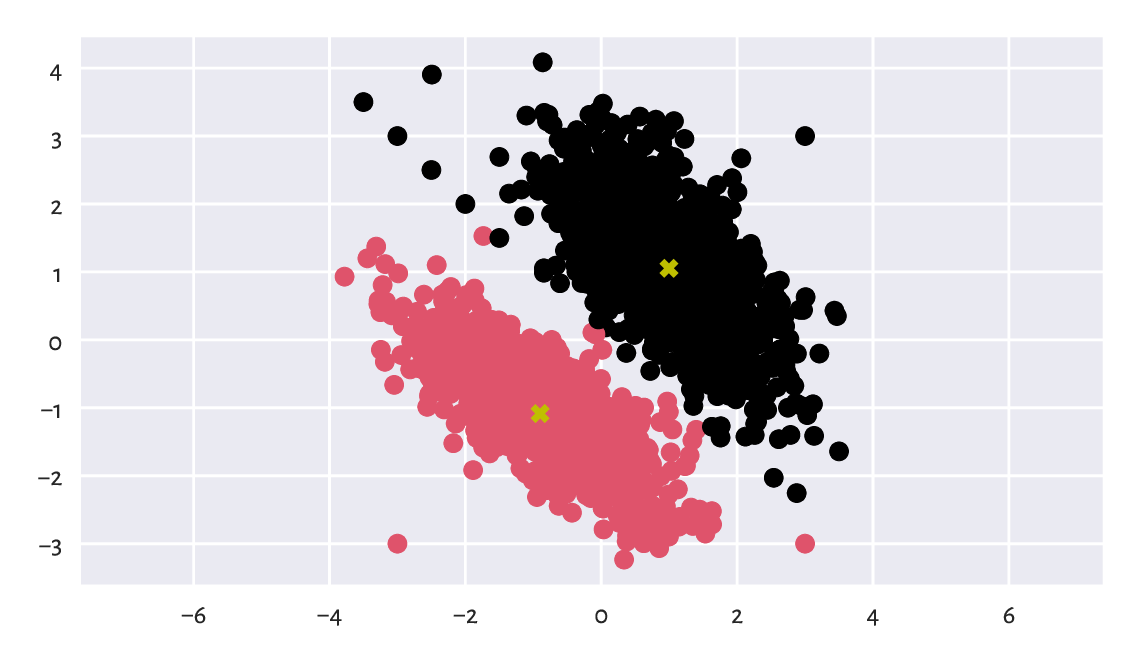Figure 12.10 The two clusters discovered by the k-means method; cluster centroids are marked in black

Here are the cluster sizes:

np.bincount(l)  # or, e.g., pd.Series(l).value_counts()
## array([1017, 1039])


The label vector l can be added as a new column in the dataset; here is a preview:

Xl = pd.DataFrame(dict(X1=X[:, 0], X2=X[:, 1], l=l))
Xl.sample(5, random_state=42)  # some randomly chosen rows
##             X1        X2  l
## 184  -0.973736 -0.417269  1
## 1724  1.432034  1.392533  0
## 251  -2.407422 -0.302862  1
## 1121  2.158669 -0.000564  0
## 1486  2.060772  2.672565  0


We can now enjoy all the techniques for processing data in groups that we have discussed so far. In particular, computing the columnwise means gives nothing else than the above cluster centroids:

Xl.groupby("l").mean()
##          X1        X2
## l
## 0  0.996230  1.052801
## 1 -0.900414 -1.084118


The label vector l can of course be recreated by referring to the distances of all the points to the centroids and picking the index of the closest pivot:

np.argmin(scipy.spatial.distance.cdist(X, C), axis=1)
## array([1, 1, 1, ..., 0, 0, 0])


Important

By construction (and its relation to Voronoi diagrams), the k-means method can only detect clusters of convex shapes (such as Gaussian blobs).

Exercise 12.19

Perform the clustering of the wut_isolation dataset and notice how nonsensical, geometrically speaking, the returned clusters are.

Note

Determine a clustering of the wut_twosplashes dataset and display the results on a scatterplot. Compare the results to those obtained on the standardised version thereof. Recall what we have said about the Euclidean distance and its perception being disturbed when a plot’s aspect ratio is not 1:1.

Note

(*) An even simpler classifier that the k-nearest neighbours one described above builds upon the concept of the nearest centroids. Namely, it first determines the centroids (componentwise arithmetic means) of the points in each class. Then, a new point (from the test set) is assigned to the class whose centroid is the closest thereto. The implementation of such a classifier is left as a rather straightforward exercise to the reader. As an application, we recommend using it to extrapolate the results generated by the k-means method to previously unobserved data.

### 12.4.2. Solving K-means is Hard

Unfortunately, the k-means method – the identification of label vectors/cluster centres that minimise the total within-cluster sum of squares – relies on solving a computationally hard combinatorial optimisation problem (e.g., [Lee11]). In other words, search for the truly (i.e., globally) optimal solution takes impractically long time for larger $$n$$ and $$k$$.

Therefore, we must rely on some approximate algorithms which all have the same drawback: whatever they return can actually be a suboptimal, and hence possibly meaningless, solution.

The documentation of scipy.cluster.vq.kmeans2 is honest about it (after all, it is a package made by people with PhDs in STEM, not working for marketing divisions of some greedy corporations), stating that the method attempts to minimise the Euclidean distance between observations and centroids. Further, sklearn.cluster.KMeans, implementing a similar algorithm, mentions that the procedure is very fast […], but it falls in local minima. That is why it can be useful to restart it several times.

To understand what it all means, it will thus be very educational to study this issue in more detail, as certainly the discussed approach to clustering is not the only hard problem in data science (selecting an optimal set of independent variables with respect to AIC or BIC in linear regression being another example).

### 12.4.3. Lloyd’s Algorithm

Technically, there is no such thing as the k-means algorithm. There many procedures, based on many different heuristics, which attempt to solve the k-means problem. Unfortunately, neither of them is of course perfect (because it is not possible).

Perhaps the most widely know (and easy to understand) method is based on fixed-point iteration and is traditionally attributed to Lloyd [Llo2)]. For a given $$\mathbf{X}\in\mathbb{R}^{n\times m}$$ and $$k \ge 2$$:

1. Pick initial cluster centres $$\boldsymbol{c}_{1}, \dots, \boldsymbol{c}_{k}$$, for example, randomly.

2. For each point in the dataset, $$\mathbf{x}_{i,\cdot}$$, determine its closest centre $$\ell_i$$:

$\ell_i = \mathrm{arg}\min_{j} \| \mathbf{x}_{i,\cdot} - \boldsymbol{c}_{j} \|^2,$
3. Compute the centroids of the clusters defined by the label vector $$\boldsymbol\ell$$, i.e., for every $$j=1,2,\dots,k$$,

$\boldsymbol{c}_j = \frac{1}{n_j} \sum_{i: \ell_i=j} \mathbf{x}_{i,\cdot},$

where $$n_j=|\{i: \ell_i=j\}|$$ gives the size of the $$j$$-th cluster.

4. If the objective function (total within-cluster sum of squares) has not changed significantly (say, the absolute value of the difference is less than $$10^{-9}$$) since the last iteration, stop and return the current $$\boldsymbol{c}_1,\dots,\boldsymbol{c}_k$$ as the result. Otherwise, go to Step 2.

Exercise 12.20

(*) Implement the Lloyd’s algorithm in the form of a function kmeans(X, C), where X is the data matrix (n-by-m) and where the rows in C, being an k-by-m matrix, give the initial cluster centres.

### 12.4.4. Local Minima

By the way the above algorithm is constructed, we have, what follows.

Important

Lloyd’s method guarantees that the centres $$\boldsymbol{c}_1,\dots,\boldsymbol{c}_k$$ it returns cannot be improved any further, at least not significantly. However, it does not necessarily mean that they yield the globally optimal (the best possible) WCSS. We might as well get stuck in a local minimum, where there is no better positioning thereof in the neighbourhood of the current cluster centres (compare Figure 12.11). Yet, had we looked beyond that, we could have found a superior solution.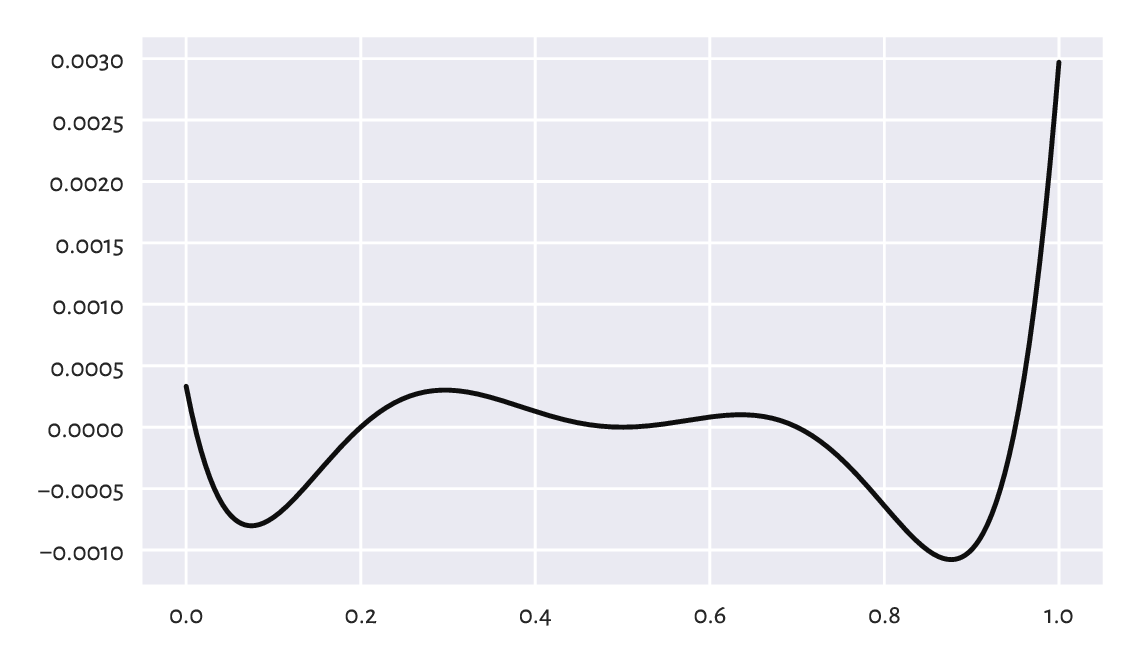Figure 12.11 An example function (of only one variable; our problem is much higher-dimensional) with many local minima; how can we be sure there is no better minimum outside of the depicted interval?

A variant of the Lloyd’s method is implemented in scipy.cluster.vq.kmeans2, where the initial cluster centres are picked at random. Let us test its behaviour by analysing three chosen country-wise categories from the 2016 Sustainable Society Indices dataset.

ssi = pd.read_csv("https://raw.githubusercontent.com/gagolews/" +
"teaching_data/master/marek/ssi_2016_categories.csv",
comment="#")
X = ssi.set_index("Country").loc[:,
["PersonalDevelopmentAndHealth", "WellBalancedSociety", "Economy"]
]
n = X.shape
X.loc[["Australia", "Germany", "Poland", "United States"], :]  # preview
##                PersonalDevelopmentAndHealth  ...   Economy
## Country                                      ...
## Australia                          8.590927  ...  7.593052
## Germany                            8.629024  ...  5.575906
## Poland                             8.265950  ...  5.989513
## United States                      8.357395  ...  3.756943
##
## [4 rows x 3 columns]


Thus, it is a three-dimensional dataset, where each point corresponds to a different country. Let us find a partition into $$k=3$$ clusters.

k = 3
np.random.seed(123)  # reproducibility matters
C1, l1 = scipy.cluster.vq.kmeans2(X, k)
C1
## array([[7.99945084, 6.50033648, 4.36537659],
##        [7.6370645 , 4.54396676, 6.89893746],
##        [6.24317074, 3.17968018, 3.60779268]])


The objective function (total within-cluster sum of squares) at the returned cluster centres is equal to:

import scipy.spatial.distance
def get_wcss(X, C):
D = scipy.spatial.distance.cdist(X, C)**2
return np.sum(np.min(D, axis=1))

get_wcss(X, C1)
## 446.5221283436733


Is it good or not necessarily? We are unable to tell. What we can do, however, is to run the algorithm again, this time from a different starting point:

np.random.seed(1234)  # different seed - different initial centres
C2, l2 = scipy.cluster.vq.kmeans2(X, k)
C2
## array([[7.80779013, 5.19409177, 6.97790733],
##        [6.31794579, 3.12048584, 3.84519706],
##        [7.92606993, 6.35691349, 3.91202972]])
get_wcss(X, C2)
## 437.51120966832775


It is a better solution (we are lucky; it might as well have been worse). But is it the best possible? Again, we cannot tell, alone in the dark.

Does a potential suboptimality affect the way the data points are grouped? It is indeed the case here. Let us take a look at the contingency table for the two label vectors:

pd.DataFrame(dict(l1=l1, l2=l2)).value_counts().unstack(fill_value=0)
## l2   0   1   2
## l1
## 0    8   0  43
## 1   39   6   0
## 2    0  57   1


Important

Clusters are actually unordered. The label vector $$(1, 1, 2, 2, 1, 3)$$ represents the same clustering as the label vectors $$(3, 3, 2, 2, 3, 1)$$ and $$(2, 2, 3, 3, 2, 1)$$.

By looking at the contingency table, we see that clusters 0, 1, and 2 in l1 correspond, respectively, to clusters 2, 0, and 1 in l2 (by a kind of majority voting). Therefore, we can relabel the elements in l1 to get a more readable result:

l1p = np.array([2, 0, 1])[l1]
pd.DataFrame(dict(l1p=l1p, l2=l2)).value_counts().unstack(fill_value=0)
## l2    0   1   2
## l1p
## 0    39   6   0
## 1     0  57   1
## 2     8   0  43


It turns out that 8+6+1 countries are categorised differently. We would definitely not want to initiate any diplomatic crisis because of our not knowing that the above algorithm might return suboptimal solutions.

Exercise 12.21

(*) Determine which countries are affected.

### 12.4.5. Random Restarts

There will never be any guarantees, but we can increase the probability of generating a good solution by simply restarting the method many times from many randomly chosen points and picking the best3 solution (the one with the smallest WCSS) identified as the result.

Let us make 1000 such restarts:

wcss, Cs = [], []
for i in range(1000):
C, l = scipy.cluster.vq.kmeans2(X, k, seed=i)
Cs.append(C)
wcss.append(get_wcss(X, C))
## /usr/local/lib/python3.9/dist-packages/scipy/cluster/vq.py:607: UserWarning: One of the clusters is empty. Re-run kmeans with a different initialization.
##   warnings.warn("One of the clusters is empty. "


The best of the local minima (no guarantee that it is the global one, again) is:

np.min(wcss)
## 437.51120966832775


It corresponds to the cluster centres:

Cs[np.argmin(wcss)]
## array([[7.80779013, 5.19409177, 6.97790733],
##        [7.92606993, 6.35691349, 3.91202972],
##        [6.31794579, 3.12048584, 3.84519706]])


Actually, it is the same as C2, l2 above (up to a permutation of labels). We were lucky, after all.

It is very educational to take a look at the distribution of the objective function at the identified local minima to see that, proportionally, in the case of this dataset it is not rare to end up in a quite bad solution; see Figure 12.12.

plt.hist(wcss, bins=100)
plt.show()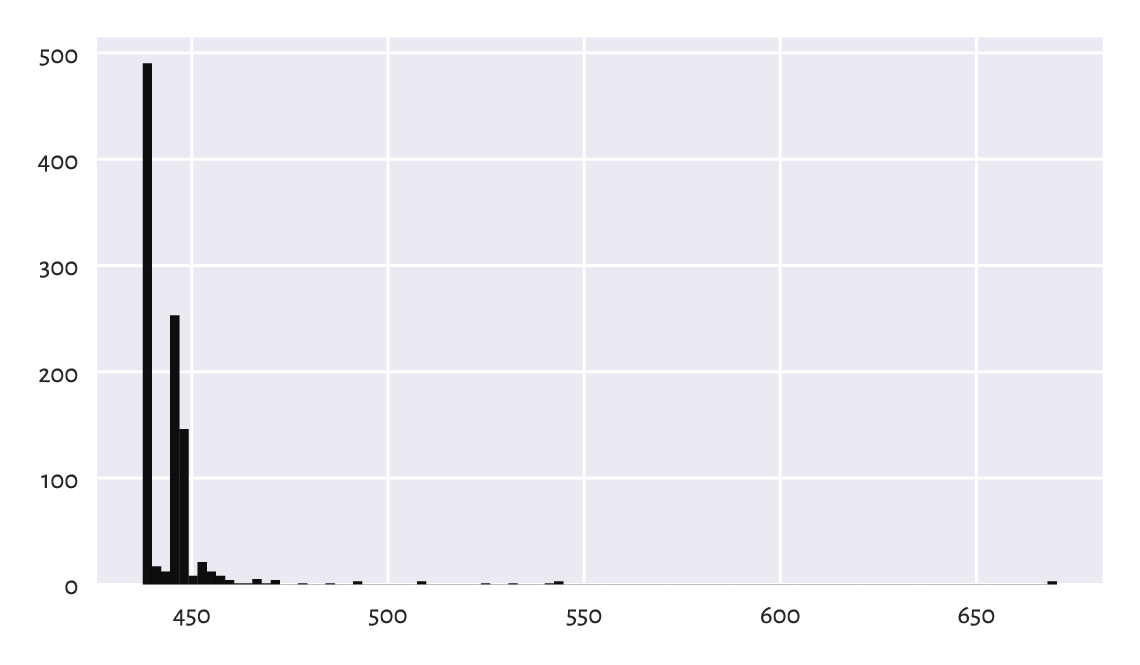Figure 12.12 Within-cluster sum of squares at the results returned by different runs of the k-means algorithm; sometimes we might be very unlucky

Also, Figure 12.13 depicts all the cluster centres that the algorithm converged to. We see that we should not be trusting the results generated by a single run of a heuristic solver to the k-means problem.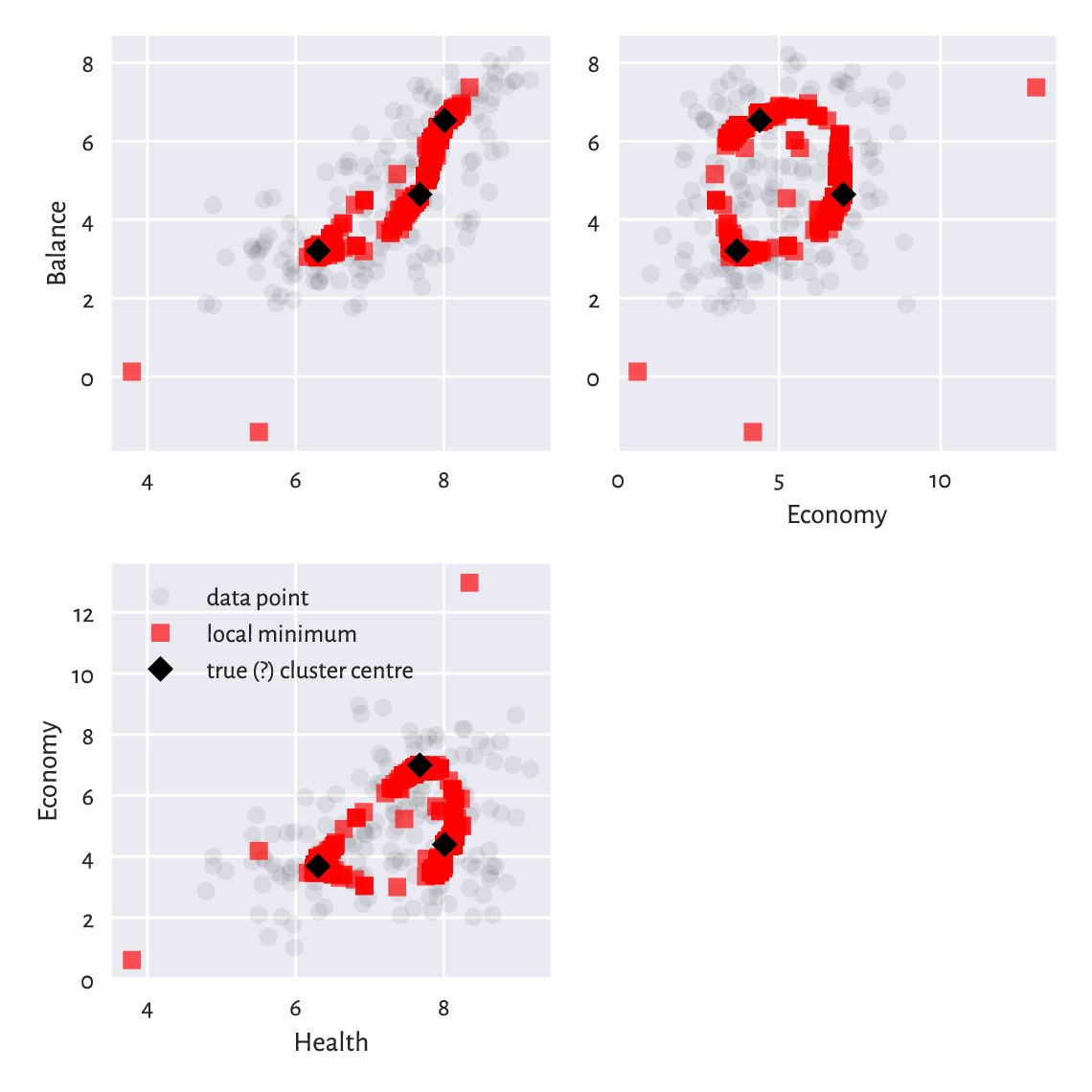Figure 12.13 Different cluster centres our k-means algorithm converged to; some are definitely not optimal, and therefore the method must be restarted a few times in order to increase the likelihood of pinpointing the true solution

Example 12.22

(*) The scikit-learn package implements an algorithm that is similar to the Lloyd’s one. The method is equipped with the n_init parameter (which defaults to 10) which automatically applies the aforementioned restarting. Still there are no guarantees:

import sklearn.cluster
np.random.seed(123)
km = sklearn.cluster.KMeans(k)  # KMeans(k, n_init=10)
km.fit(X)
## KMeans(n_clusters=3)
km.inertia_  # WCSS
## 437.5467188958929


In this case, the solution is suboptimal too. As an exercise, we suggest the reader to pass n_init=100, n_init=1000, and n_init=10000 and determine the best WCSS.

Note

It is theoretically possible that a developer from the scikit-learn team, when they see the above result, will make a tweak in the algorithm so that after an update to the package, the returned minimum will be better. This cannot be deemed a bug fix, though, as there are no bugs here. Improving the behaviour of the method on this example will lead to its degradation on others. There is no free lunch in optimisation.

Note

Some datasets are more well-behaving than others. The k-means method is overall quite usable, but we should always be cautious.

Exercise 12.23

Run the k-means method, $$k=8$$, on the sipu_unbalance dataset numerous times. Note the value of the total within-cluster sum of squares. Also, plot the cluster centres discovered. Do they make sense? Compare these to the case where you start the method from the following cluster centres which are close to the global minimum.

$\begin{split} \mathbf{C} = \left[ \begin{array}{cc} -15 & 5 \\ -12 & 10 \\ -10 & 5 \\ 15 & 0 \\ 15 & 10 \\ 20 & 5 \\ 25 & 0 \\ 25 & 10 \\ \end{array} \right] \end{split}$

An overall good introduction to classification is [HTF17] and [Bis06]. However, as we have said earlier, we recommend going through a solid course in matrix algebra and probability and statistics first, e.g., [DFO20, Gen17] and [DKLM05, Gen09, Gen20].

For advanced theoretical (probabilistic, information-theoretic) results, see, e.g., [BHK20, DGL96].

## 12.6. Exercises

Exercise 12.24

Name the data type of an object that the DataFrame.groupby method returns.

Exercise 12.25

What is the relationship between GroupBy, DataFrameGroupBy, and SeriesGroupBy?

Exercise 12.26

What are relative z-scores and how can we compute them?

Exercise 12.27

Why and when the accuracy score might not be the best way to quantify a classifier’s performance?

Exercise 12.28

What is the difference between recall and precision, both in terms of how they are defined and where they are the most useful?

Exercise 12.29

Explain how a k-nearest neighbour classification algorithm works. Why do we say that is is model-free?

Exercise 12.30

In the context of k-nearest neighbour classification, why it might be important to resolve the potential ties at random when computing the mode of the neighbours’ labels?

Exercise 12.31

What is the purpose of a train-test and train-validation-test set splits?

Exercise 12.32

What is the difference between the fixed-radius and few-nearest-neighbours search?

Exercise 12.33

Give the formula for the total within-cluster sum of squares.

Exercise 12.34

Are there any cluster shapes that cannot be detected by the k-means method?

Exercise 12.35

Why do we say that solving the k-means problem is hard?

Exercise 12.36

Why restarting Lloyd’s algorithm a few times is usually necessary?

1

Including those that are merely due to round-off errors, in very large samples.

2

Of course we do not have to denote the number of clusters with k: we could be speaking about the 2-means, 3-means, c-means, or ę-means method too. Nevertheless, some mainstream practitioners consider k-means as a kind of a brand name, let us thus refrain from adding to their confusion.

3

If we have many different heuristics, each aiming to approximate a solution to the k-means problem, from the practical point of view it does not really matter which one returns the best solution – they are merely our tools to achieve a higher goal. Ideally, we should run all of them a few times and just get the result that corresponds to the smallest WCSS. What matters is that we have did our best to find the optimal set of cluster centres – and of course more approaches tested improve the chance of success.